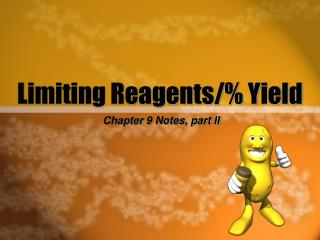DownloadDownload PresentationLimiting Reagents/% Yield

# Limiting Reagents/% Yield

Download Presentation## Limiting Reagents/% Yield

- - - - - - - - - - - - - - - - - - - - - - - - - - - E N D - - - - - - - - - - - - - - - - - - - - - - - - - - -
##### Presentation Transcript

1. Limiting Reagents/% Yield Chapter 9 Notes, part II

2. What are limiting reagents? • Up until now, we have assumed that all reactants are used up in a reaction. • In actuality, usually one chemical runs out first, stopping the reaction—that chemical is called the limiting reagent.

3. The limiting reagent limits or determines the amount of product that can be formed in a reaction. On the flip side, the excess reagent is the one not completely used up.

4. How do you find what the limiting reagent is? • Set up as many sets of dimensional analysis as pieces of data you are given. • Solve each, and the one with the lowest amount of product formed is the correct answer. • The chemical you started with is the limiting reagent.

5. Here’s an example: In the reaction Mg + HCl a MgCl2 + H2 you combine 35.5 grams of magnesium with 28.1 grams of hydrochloric acid. Which one is the limiting reagent, and how much magnesium chloride is produced?

6. Percent Yield • In a real world setting, you never form 100% of the products that you think you should based on theoretical calculations. • By comparing how much of a product forms and how much should formed based on stoichiometry, you can find the percent yield.

7. Percent Yield • %Yield= actual yield x100 theoretical yield

8. Practice Problem • In the reaction of nitrogen gas and hydrogen gas producing ammonia (NH3), 250.2g H2 produces 1095.1g NH3. What is the % yield of this reaction?

9. Practice Problem • K2CrO4 + Pb(NO3)2a KNO3 + PbCrO4 • If 0.947g K2CrO4 are combined with 0.331g Pb(NO3)2 what will be the limiting reagent, and how many grams of lead chromate is produced?

10. Practice Problem • Zn + HCl a ZnCl2 + H2 • What is the limiting reagent and how many liters of hydrogen are produced if 15.5 grams of zinc are combined with 26.3 grams of hydrochloric acid?

11. Tougher Practice Problem • If 12.2mL of ethanol (C2H5OH) are added to 3.78L of O2 and the ethanol undergoes combustion, how many milliliters of water are formed? Density of water is 1.00g/mL and density of ethanol is 0.779g/mL.

12. Practice Problem • In the reaction: Mg3N2 + 6H2O a 3Mg(OH)2 + 2NH3 • If the initial amount of Mg3N2 is 58.1g and the initial amount of H2O is 20.4g, and 26.2g of Mg(OH)2 are produced, what is the percent yield of the reaction?

13. In Review: What is a limiting reagent? What is the excess reagent? How does a limiting reagent control the amount of product? What is percent yield?